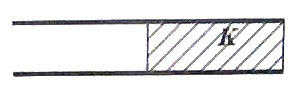## Pages

`“Life is like riding a bicycle.  To keep your balance you must keep moving.”–Albert Einstein`

## Tuesday, July 22, 2008

### AP Physics B & C – Electrostatics – Multiple Choice Questions on Capacitors

Here are some typical multiple choice questions (for practice) on capacitors:

(1) Capacitors C1, C2 and C3 of values 15 μF, 10 μF, 3 μF are connected in series and the series combination is connected across a battery of emf 10 V. When the capacitors are fully charged, the charge on one plate of the 3 μF capacitor will be of magnitude

(a) 10 μC

(b) 15 μC

(c) 3 μC

(d) 20 μC

(e) 280 μC

The series combined value (C) of the three capacitors is given by

1/C = 1/C1 + 1/C2 + 1/C3 = 1/15 + 1/10 + 1/3 so that C = 2 μF

The charge (Q) on this equivalent capacitance is given by

Q=CV = 2 μF ×10 V = 20 μC

When capacitors are connected in series, the charges on the plates of all capacitors are of the same magnitude so that the correct option is 20 μC [Option (d)].

(2) A 2 μF capacitor connected in a circuit has one plate at + 6 V and the other plate at – 6 V. The charge on the negative plate of the capacitor is

(a) + 12 μC

(b) 12 μC

(c) + 24 μC

(d) 24 μC

(e) zero

The magnitude of charge on either plate is given by

Q = CV

Note that V is the potential difference between the plates and is equal to 6 – (– 6) = 12 V.

Therefore, Q = 2 μF × 12 V = 24 μC

Since the charge on the negative plate must be negative, the answer is 24 μC.

(3) A parallel plate capacitor with air as dielectric remains connected across a battery of emf 6 V. The charge on the capacitor in this condition is Q. If the separation between the plates is decreased by 10% in this condition and sufficient time is allowed to attain steady state, the charge on the capacitor will be

(a) unchanged

(b) increased by 9%, approximately

(c) decreased by 9%, approximately

(d) decreased by 11%, approximately

(e) increased by 11%, approximately

The correct option is (e) since the capacitance will increase by approximately 11% in accordance with the expression,

C = ε0A/d when the separation d changes to 0.9d.

[Remember that Q = CV]

Now, note the following in connection with the above question:

If the battery is disconnected after charging the capacitor to have an initial charge Q,

(i) the charge on the plates will be unchanged if the plate separation is decreased or increased (since there is no battery to control the charge). The law of conservation of charge is very strictly obeyed.

(ii) The potential difference between the plates of the capacitor will be decreased on decreasing the separation between the plates since V = Q/C and Q is unchanged where as C is increased.

(iii) The potential difference between the plates of the capacitor will be increased on increasing the separation between the plates since V = Q/C and Q is unchanged where as C is decreased.

(iv) If a dielectric slab is inserted in to the gap between the plates, the potential difference between the plates will be decreased since the capacitance is increased (with the charge on the plates unchanged).

* * * * * * * * * * * * * *

(4) A parallel plate capacitor with air as dielectric remains connected across a battery. After the capacitor is fully charged in this condition, a slab of dielectric constant 5 is slowly introduced into the gap between the plates. Which one of the following statements is true during the introduction of the slab?

(a) The charge on the capacitor will gradually decrease

(b) The capacitance will gradually decrease

(c) A current will flow through the leads connecting the capacitor to the battery

(d) The potential difference between the plates of the capacitor will gradually increase

(e) The potential difference between the plates of the capacitor will gradually decrease

When the slab is introduced, the capacitance gradually increases and the battery supplies more charges to the capacitor since the charge Q has to increase in accordance with the equation, Q = CV. The potential difference across the capacitor will be unchanged since it is connected across the battery (whose emf is fixed).

The only correct option therefore is (c).

(5) Half of the space between the plates of a parallel plate air capacitor of capacitance C is filled as shown with a material of dielectric constant K. The new capacitance will be(a) KC

(b) KC/2

(c) (K + 1)C/2

(d) 2C/K

(e) (K 1)C

Initially the capacitance C is given by

C = ε0A/d where A and d are the plate area and plate separation respectively.

On introducing the dielectric material, you can treat the new capacitor to be made of two capacitors, one with air as dielectric and the other with the introduced material as the dielectric. But the area of each capacitor is half that of the full capacitor. Further, the two capacitors are in parallel and hence the capacitance (C’) of the new capacitor is given by

C’ = ε0A/2d + Kε0A/2d

Putting C = ε0A/d, we obtain C’ = (K + 1)C/2.

We will discuss more questions on capacitors in due course. Meanwhile, find some useful and interesting multiple choice questions (with solution) at physicsplus.

1.2.